Courses

Ex 6.1 NCERT Solutions- Lines and Angles Notes | Study Class 9 Mathematics by VP Classes - Class 9

Class 9: Ex 6.1 NCERT Solutions- Lines and Angles Notes | Study Class 9 Mathematics by VP Classes - Class 9

The document Ex 6.1 NCERT Solutions- Lines and Angles Notes | Study Class 9 Mathematics by VP Classes - Class 9 is a part of the Class 9 Course Class 9 Mathematics by VP Classes.
All you need of Class 9 at this link: Class 9

Q.1. In the following figure,  lines AB and CD intersect at O. If AOC +BOE = 70° and BOD = 40°, find BOE and reflex COE.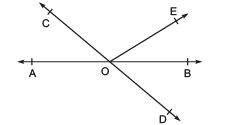Solution:

From the diagram, we have
(∠AOC +∠BOE +∠COE) and (∠COE +∠BOD +∠BOE) forms a straight line.
So, ∠AOC+∠BOE +∠COE = ∠COE +∠BOD+∠BOE = 180°
Now, by putting the values of ∠AOC + ∠BOE = 70° and ∠BOD = 40° we get
∠COE = 110° and ∠BOE = 30°
So, reflex ∠COE = 360° – 110° = 250°

Q.2. In the following figure, lines XY and MN intersect at O. If POY = 90° and a : b = 2 : 3, find c.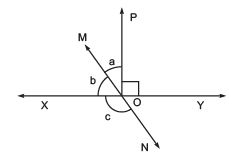Solution:
We know that the sum of linear pair are always equal to 180°
So,
POY +a +b = 180°
Putting the value of POY = 90° (as given in the question) we get,
a+b = 90°
Now, it is given that a : b = 2 : 3 so,
Let a be 2x and b be 3x
∴ 2x+3x = 90°
Solving this we get
5x = 90°
So, x = 18°
∴ a = 2×18° = 36°
Similarly, b can be calculated and the value will be
b = 3×18° = 54°

From the diagram, b+c also forms a straight angle so,
b+c = 180°
c+54° = 180°
∴ c = 126°

Q.3. In the following figure, PQR = PRQ, then prove that PQS = PRT.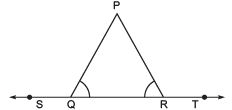Solution:
Since ST is a straight line so,
∠PQS+∠PQR = 180° (linear pair) and
∠PRT+∠PRQ = 180° (linear pair)
Now, ∠PQS + ∠PQR = ∠PRT+∠PRQ = 180°
Since ∠PQR =∠PRQ (as given in the question)
∠PQS = ∠PRT. (Hence proved).

Q.4. In the following figure, if x+y = w+z, then prove that AOB is a line.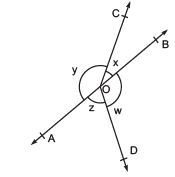Solution:
For proving AOB is a straight line, we will have to prove x+y is a linear pair
i.e. x+y = 180°
We know that the angles around a point are 360° so,
x+y+w+z = 360°
In the question, it is given that,
x+y = w+z
So, (x+y)+(x+y) = 360°
2(x+y) = 360°
∴ (x+y) = 180° (Hence proved).

Q.5. In the adjoining figure, POQ is a line. Ray OR is perpendicular to line PQ. OS is another ray lying between rays OP and OR. Prove that ROS = ½ (QOS – POS).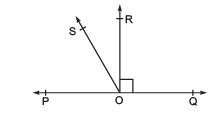Solution:
In the question, it is given that (OR ⊥ PQ) and POQ = 180°
So, POS+ROS+ROQ = 180°
Now, POS+ROS = 180°- 90° (Since POR = ROQ = 90°)
∴ POS + ROS = 90°
Now, QOS = ROQ+ROS
It is given that ROQ = 90°,
∴ QOS = 90° +ROS
Or, QOS – ROS = 90°
As POS + ROS = 90° and QOS – ROS = 90°, we get
POS + ROS = QOS – ROS
2 ROS + POS = QOS
Or, ROS = ½ (QOS – POS) (Hence proved).

Question 6. It is given that XYZ = 64° and XY is produced to point P. Draw a figure from the given information. If ray YQ bisects ZYP, find XYQ and reflex QYP.
Solution: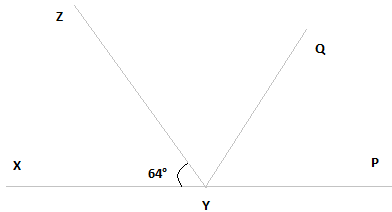Here, XP is a straight line
So, XYZ +ZYP = 180°
Putting the value of XYZ = 64° we get,
64° +ZYP = 180°
∴ ZYP = 116°
From the diagram, we also know that ZYP = ZYQ + QYP
Now, as YQ bisects ZYP,
ZYQ = QYP
Or, ZYP = 2ZYQ
∴ ZYQ = QYP = 58°
Again, XYQ = XYZ + ZYQ
By putting the value of XYZ = 64° and ZYQ = 58° we get.
XYQ = 64°+58°
Or, XYQ = 122°
Now, reflex QYP = 180°+XYQ
We computed that the value of XYQ = 122°.
So,
QYP = 180°+122°
∴ QYP = 302°

The document Ex 6.1 NCERT Solutions- Lines and Angles Notes | Study Class 9 Mathematics by VP Classes - Class 9 is a part of the Class 9 Course Class 9 Mathematics by VP Classes.
All you need of Class 9 at this link: Class 9Use Code STAYHOME200 and get INR 200 additional OFF Use Coupon Code

Top Courses for Class 9Class 9 Mathematics by VP Classes

125 docs

Top Courses for Class 9Track your progress, build streaks, highlight & save important lessons and more!

,

,

,

,

,

,

,

,

,

,

,

,

,

,

,

,

,

,

,

,

,

;